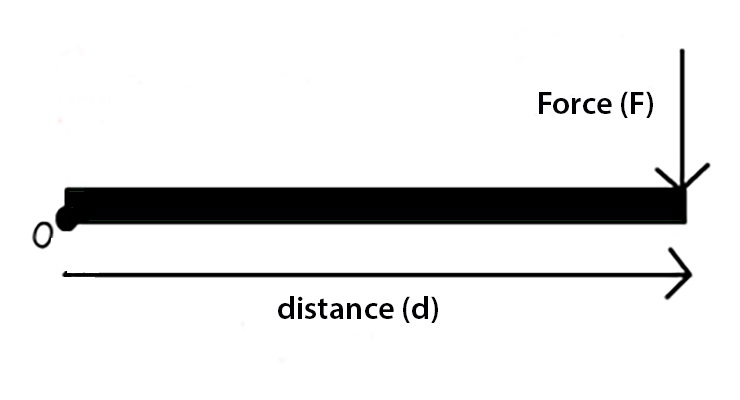# Moment Formula

## Moment Formulas - Definitions, Equation & Examples

The turning effect of a force is known as the moment. It is the product of the force multiplied by the perpendicular distance from the line of action of the force to the pivot or point where the object will turn.

SI unit of moment of a force is Newton-metre (Nm). It is a vector quantity.
Its direction is given by the right-hand grip rule perpendicular to the plane of the force and pivot point which is parallel to the axis of rotation.𝝉=F×d

where 𝝉 is the moment of force/torque
F is the force
d is the perpendicular distance from the line of action of the force to the pivot

Example:
The moment of force of 10 N about a point is 3 Nm. The distance of point of application of the force from the point is
Moment of force = force × distance
⇒ 3 Nm = 10 N × r
⇒ r = 0.3 m

Question:
If a uniform bar of mass 8 Kg is pivoted at one end, What is the net moment of force on the bar.